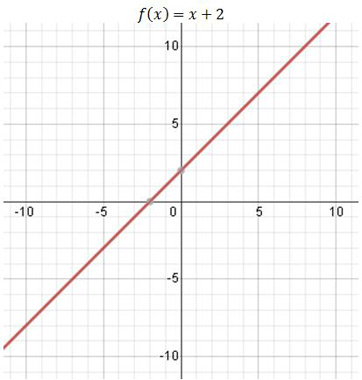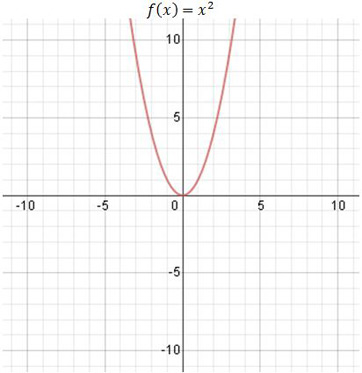# One-to-One Functions: Definitions and Examples

An error occurred trying to load this video.

Try refreshing the page, or contact customer support.

Coming up next: How to Determine the Limits of Functions

### You're on a roll. Keep up the good work!

Replay
Your next lesson will play in 10 seconds
• 0:02 A Function
• 1:01 A One-to-One Function
• 1:39 Graphs
• 2:31 The Horizontal Line Test
• 3:12 Lesson Summary
Save Save

Want to watch this again later?

Timeline
Autoplay
Autoplay
Speed Speed

#### Recommended Lessons and Courses for You

Lesson Transcript
Instructor: Yuanxin (Amy) Yang Alcocer

Amy has a master's degree in secondary education and has taught math at a public charter high school.

Watch this video lesson to learn what makes a one-to-one function different from a regular function. Learn a simple test you can use to check whether a function is one-to-one or not.

## A Function

A good way of describing a function is to say that it gives you an output for a given input. You give functions a certain value to begin with and they do their thing on the value, and then they give you the answer. For example, the function f(x) = x + 1 adds 1 to any value you feed it. You give it a 5, this function will give you a 6: f(5) = 5 + 1 = 6.

Functions do have a criterion they have to meet, though. And that is the x value, or the input, cannot be linked to more than one output or answer. In other words, you cannot feed the function one value and end up with two different answers. For example, if you give a supposed function a 1 and it gives you a 4 and a 10, then you know that this supposed function is not a real function. A real function would give you one solid answer only.

## A One-to-One Function

Now, let's talk about one-to-one functions. A one-to-one function is a function in which the answers never repeat. A normal function can have two different input values that produce the same answer, but a one-to-one function does not. For example, the function f(x) = x^2 is not a one-to-one function because it produces 4 as the answer when you input both a 2 and a -2, but the function f(x) = x - 3 is a one-to-one function because it produces a different answer for every input.

## Graphs

We can learn a lot by comparing graphs of functions that are and are not one-to-one functions. Let's look at one that is and one that is not a one-to-one function.

The function shown here is f(x) = x + 2, and it is a one-to-one function.You can see that every input, x, produces a different answer, y.

Now, let's look at the graph of f(x) = x^2, which is not a one-to-one function.This function is not a one-to-one function because we have two different input values, x, that produce the same answer, y. Look at the graph when the input is both a 3 and a -3. You can see that both produce 9 as the answer.

To unlock this lesson you must be a Study.com Member.

### Register to view this lesson

Are you a student or a teacher?

#### See for yourself why 30 million people use Study.com

##### Become a Study.com member and start learning now.
Back
What teachers are saying about Study.com

### Earning College Credit

Did you know… We have over 200 college courses that prepare you to earn credit by exam that is accepted by over 1,500 colleges and universities. You can test out of the first two years of college and save thousands off your degree. Anyone can earn credit-by-exam regardless of age or education level.